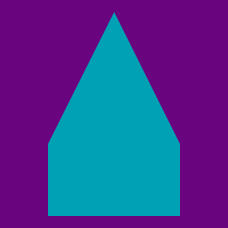Geometry

# General Polygons - Area

What is the area of the shaded hexagon in rectangle $ABCD$ in the diagram below?Details and assumptions:

• If your answer is $12 \text{ cm}^2$, type it in as $12$.

What is the area of hexagon $ABCDEF$ in the diagram below?Details and assumptions:

• Let $\sqrt{3}=1.732.$
• In the diagram above, red lines are parallel to one another and blue lines are parallel to one another.

What is the area of quadrilateral $ABCD$ in the diagram below?Details and assumptions:

• Let $\sqrt{3}=1.732.$

What is the area of the nonagon inscribed in the circle with radius 4 below?Details and assumptions:

• Use the following formula to find $\sin 15^\circ :$ $\sin^2 15^\circ = \dfrac {1-\cos 30^\circ}{2}.$
• Let $\sqrt{2}=1.414,$ $\sqrt{3}=1.732,$ and $\sqrt{6}=2.449.$

What is the area of the regular hexagon below, where the area of the shaded region is 34?×